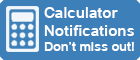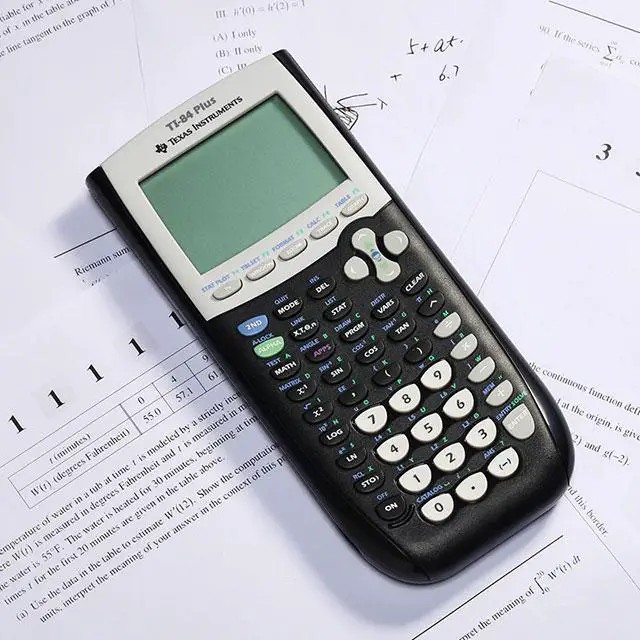Calculator Library
Free Online CalculatorsHome » 87 Calculators » Blog » Texas Instruments TI-84 Calculator

Huge Domain Name Sale | Free Domains
Domain names on sale at crazy prices. Register a new domain for FREE when you buy another service.

## TEXAS INSTRUMENTS TI-84 CALCULATORThe Texas Instruments TI-84 calculator is a popular graphing calculator used by students and professionals alike and continues to be a valuable tool for solving complex problems and performing advanced calculations.

With its easy-to-use interface and powerful capabilities, it has become a staple in the educatonal market and is used extensively by students in school, college and university environments.

## TI-84 Calculator History

The TI-84 calculator has a rich history dating back to the 1980s, when Texas Instruments (TI) first introduced the TI-81, a basic graphing calculator.

In 1990, Texas Instruments released the TI-82, an upgraded version of the TI-81 with additional features such as basic programming capabilities and the ability to solve equations. The TI-83, released in 1992, built on the success of the TI-82 and added more advanced graphing and programming capabilities.

Released in 1996, the TI-84 was a major step forward for TI calculators. It featured a more powerful processor, a larger screen and increased memory for storing programs and data. It also had the ability to graph and analyze functions, as well as perform basic statistical calculations.

The TI-84 was a huge success and quickly became the go-to calculator for students taking advanced math and science courses. It was also widely used by professionals in fields such as engineering and finance. In addition to its powerful computational abilities, the TI-84 also had a user-friendly interface and was easy to use.

Over the years, TI has released several updated versions of the TI-84, including the TI-84 Plus and the TI-84 Plus Silver Edition. These updates added new features and improved upon the original design, making the TI-84 even more powerful and versatile.

## TI-84 Calculator Features

### Color Screen

One of the standout features of the TI-84 is its color screen, which allows for easy reading and visualization of graphs and equations. This is especially useful in math and science classes, where graphing is an essential part of the curriculum.

In addition to its graphing capabilities, the TI-84 also offers a range of functions for performing advanced calculations. This includes tools for calculus, statistics and probability, as well as a variety of scientific and engineering functions.

### Built-In Library

The TI-84 also has a built-in library of functions, which can be accessed by pressing the "2nd" button and then selecting the appropriate function from the menu. This can save time and effort for students, who no longer need to enter complex equations manually.

### Data Storage

Another useful feature of the TI-84 is its ability to store and recall data. This allows students to enter and save multiple sets of data, which can be easily accessed and used in subsequent calculations. This is especially useful in science experiments, where students can save data from each trial and then use it to compare and analyze their results.

### Ease Of Use

Despite its advanced capabilities, the TI-84 is relatively easy to use. It has a straightforward interface and comes with a user manual that provides step-by-step instructions for performing various calculations. Additionally, there are many online resources and tutorials available that can help students learn how to use the TI-84 effectively.

### Price

One potential downside of the TI-84 is its price, and it can be relatively expensive compared to other calculators on the market. However, many schools and universities require the use of a TI-84 for certain classes, so students may have no choice but to purchase one.

## How To Use The TI-84 Calculator

### Calculator Keys

Some of the most commonly used keys on the TI-84 include:

1. ON turns the calculator on and off.
2. CLEAR clears the calculator's memory and resets any settings.
3. ENTER enters values and confirms selections.
4. MODE allows you to change the settings on the calculator, such as the number of decimals displayed or the angle units used for trigonometric functions.
5. navigate through the menus and select the desired function.
6. Numbers and expressions are entered using the keypad, in the same way you would write them on paper. For example, to add 3 and 4, enter 3 + 4 and press ENTER.
7. MATH accesses the calculator's built-in math functions and programs such as square roots, sines and cosines.
8. GRAPH opens the graphing menu and allows you to graph equations and functions.
9. TABLE opens the table menu and allows you to create tables of values for equations and functions.
10. WINDOW opens a menu to set the range and scale of the graphs and tables.
11. ZOOM opens a menu to zoom in or out of graphs and adjust the viewing window.
12. APPS opens a menu where you can browse and download a variety of apps and games.
13. TRACE traces a graph and displays information about points on the graph.
14. VARS accesses and manipulates variables.
15. 2ND accesses the secondary functions of other keys. For example, pressing 2ND followed by SIN accesses the inverse sine function.

### Operation

To use the TI-84 calculator, you first need to become familiar with its keypad and layout. The calculator has a large, color LCD screen that displays the results of your calculations, as well as any graphs or equations you have entered. The keypad has a standard arrangement of numeric keys, as well as a variety of function keys that are used to access different calculator features.

One of the most useful functions of the TI-84 calculator is its ability to graph equations and data. To graph an equation, you first need to enter the equation into the calculator. To do this, press the Y= button and then use the numeric keys and function keys to enter the equation. For example, if you want to graph the equation y=x^2, you would press Y=, followed by x^2 and then ENTER.

Once you have entered the equation, you can press the GRAPH button to see a graph of the equation on the screen. The graph will be displayed using the default window settings, which show the X and Y axes with a range of -10 to 10. You can change the window settings by pressing the ZOOM button and then selecting a different option from the menu that appears. For example, you can use the ZoomFit option to automatically adjust the window settings to fit the graph on the screen.

In addition to graphing equations, the TI-84 calculator can also be used to perform a wide range of mathematical operations. For example, you can use the calculator to solve equations, find the value of a variable or calculate the mean, median and mode of a set of data. To access these functions, press the MATH button to open a menu of mathematical operations that you can perform. Use the arrow keys to highlight the operation you want to use and then press ENTER to perform the operation.

Another useful feature of the TI-84 calculator is its ability to store and recall data and equations. This can be helpful if you need to use the same data or equation multiple times in a calculation. To store a value or equation, press the STO> button. This will open a menu where you can select a location to store the value. Use the arrow keys to highlight the location you want to use, then press ENTER to store it.

To recall a stored value or equation, press the 2nd button followed by the STO> button and then select the memory location where the value is stored. It will be inserted into the current calculation, where you can use it just like any other value or equation.

In addition to these basic features, the TI-84 calculator has many other functions and capabilities that you can explore and use. For example, the calculator has a built-in programming language that you can use to create custom programs and functions. It also has a variety of advanced math and science functions, such as trigonometric and statistical functions, that you can use to solve complex problems.

To learn more about the full range of features and capabilities of the TI-84 calculator, you can refer to the user manual or visit the Texas Instruments website.

In conclusion, the TI-84 is a powerful and versatile calculator that's well-suited for a wide range of academic subjects. Its color screen, advanced functions and easy-to-use interface make it a valuable tool for students at all levels. Although it may be on the pricier side, the TI-84 is worth the investment for anyone in need of a high-quality calculator.

 Previous Post« What Is A Non-Programmable Calculator? Next PostCasio fx-350MS Calculator »